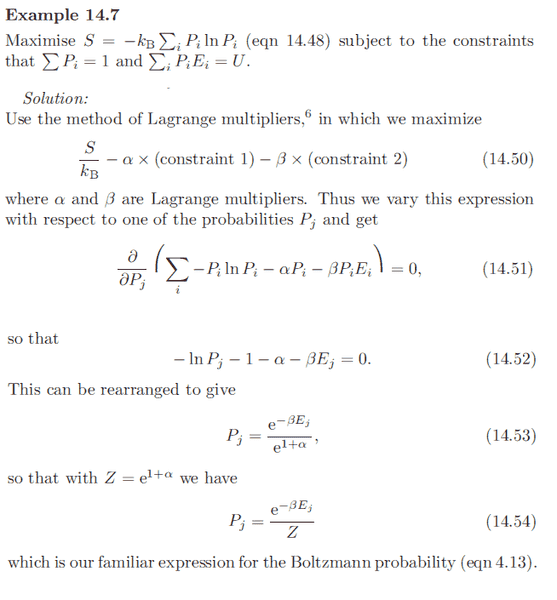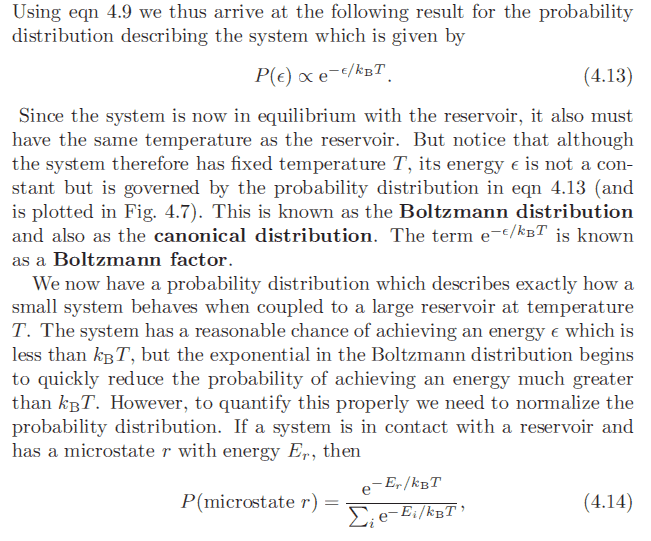# Maxwell-Boltzmann Distribution and Probability

unscientific
In the book 'Macroscopic and Statistical Thermodynamics' they derived the Maxwell-Boltzmann distribution by maximizing entropy using lagrangian multipliers with constants ##\alpha## and ##\beta##.

The final result is given as:

$$\frac{\overline {n_j}}{g_j} = e^{-\beta \epsilon_j}e^{-\alpha}$$

where ##\overline {n_j}## is the occupation number and ##g_j## is the number of states of jth energy level.

After solving for ##e^{-\alpha} = \frac{N}{V}\left(\frac{h^2}{2\pi mkT}\right)^{\frac{3}{2}}## and integrating density of states to find ##g_j = \frac{V}{h^3} 4\pi p^2 dp##:

We obtain the maxwell-boltzmann distribution:

$$\overline {n_j} = \overline {n_{(p)}} dp = N 4\pi \left(\frac{\beta}{2\pi m}\right)^{\frac{3}{2}} p^2 e^{\frac{-p^2}{2mkT}} dp$$

I obtain the correct speed distribution ##\propto p^2 e^{\frac{-p^2}{2mkT}}##, but What is the probability/fraction of finding a particle with momentum p?

In Blundell's Book, a shorter approach is taken using gibbs' expression for entropy to find the boltzmann probability:Here's the earlier reference to equation (4.13):Last edited:
•1 person

## Answers and Replies

Einj
Since the momentum p is a continuous quantity, looking for the probability of finding a particle with exactly p is pretty meaningless. One usually says that, if $f(p)$ is the Maxwell-Boltzman distribution then the probability of find a particle with momentum between p and p+dp is:
$$P_{[p,p+dp]}=f(p)dp.$$
For a general interval $p\in[p_1,p_2]$ the probability is simply:
$$P_{[p_1,p_2]}=\int_{p_1}^{p_2}f(p)dp.$$

unscientific
Since the momentum p is a continuous quantity, looking for the probability of finding a particle with exactly p is pretty meaningless. One usually says that, if $f(p)$ is the Maxwell-Boltzman distribution then the probability of find a particle with momentum between p and p+dp is:
$$P_{[p,p+dp]}=f(p)dp.$$
For a general interval $p\in[p_1,p_2]$ the probability is simply:
$$P_{[p_1,p_2]}=\int_{p_1}^{p_2}f(p)dp.$$

Yes I get all that, but what is ##f_{(p)}## from ##\frac{\overline {n_j}}{g_j} = e^{-\beta \epsilon_j}e^{-\alpha}##?

Einj
Well, I suppose that $\bar{n}_j=\bar{n}(p)dp$ is the average number of particles with momentum between p and p+dp. As you can see from your expression it is equal to the total number of particles $N$ times something. By definition, that "something" is your distribution $f(p)$.

dauto
Just divide by N.
$$f=\frac n N$$

unscientific
Well, I suppose that $\bar{n}_j=\bar{n}(p)dp$ is the average number of particles with momentum between p and p+dp. As you can see from your expression it is equal to the total number of particles $N$ times something. By definition, that "something" is your distribution $f(p)$.

Ok, so the momentum distribution is:

$$\overline {n_j} = \overline {n}_{(\vec {p})} d^3p = N \left(\frac{\beta}{2\pi m}\right)^{\frac{3}{2}} e^{\frac{-p^2}{2mkT}} d^3\vec{p}$$

By changing the dp's to dv's, thus the velocity distribution is:

$$\overline {n_j} = \overline {n}_{(\vec {v})} d^3v = N \left(\frac{\beta m}{2\pi }\right)^{\frac{3}{2}} e^{\frac{-p^2}{2mkT}} d^3\vec{v}$$

For a particular direction ##v_x##, simply just take one part of 'dv':

$$\overline {n_j} = \overline {n}_{(\vec {v_x})} dv_x = N \left(\frac{\beta m}{2\pi }\right)^{\frac{1}{2}} e^{\frac{-p_x^2}{2mkT}} d\vec{v}_x$$

With ##f_{(\vec{v})} = \frac{n_j}{N} = \left(\frac{\beta m}{2\pi}\right)^{\frac{3}{2}} e^{\frac{-p^2}{2mkT}}## and

##f_{(\vec{v_x})} = \left(\frac{\beta m}{2\pi}\right)^{\frac{1}{2}} e^{\frac{-p_x^2}{2mkT}}##

To change from velocity to speed distribution simply expand the ##d^3\vec{p}## to ##4\pi p^2 dp## or ##d\vec{v_x} = 4\pi v^2_x dv_x##.

Last edited:
Einj
At a first glance I would say that all the factors are correct. So, yes!

•1 person